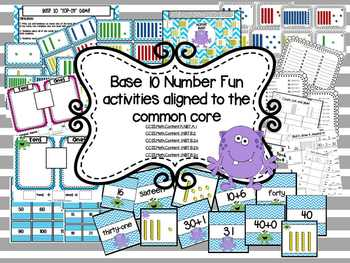# Base 10 and Place Value Math Centers and GamesResource Type
Format
PDF (13 MB|54 pages)
Standards
\$4.00
\$4.00

### Description

These base 10 math centers are filled with different games and math centers to help students understand place value. These math centers will help children compose and decompose numbers while demonstrating their understanding for each digit.

To help students develop an understanding during guided math groups:
Expanded Form Printable to introduce decomposing numbers.
Math Mats (for tens and ones as well as for expanded notation.)
Number Cards to use to help students make 2 digit numbers.
Math Centers:
30 Task Cards and 3 ways to use the cards (tens and ones practice, expanding number practice and counting base 10 Blocks.)
Over 120 matching cards for students to recognizing and become familiar with seeing numbers in different forms (words, number, expanded number, and using base 10 blocks.)
Math Center Games: Base 10 Top-It which is played similar to war with base 10 block cards and Monster Bump!
Printables: to hold students accountable during math centers, to be used as homework, or to be used as an assessment.

I hope your classroom can enjoy these base 10 and place value math center activities as much as mine does! Please note that this activity pack is alined to the common core for both kindergarten and first grade math. However, this pack was made with my first graders in mind!

Kindergarten Alignment:
CCSS.Math.Content.K.NBT.A.1 Compose and decompose numbers from 11 to 19 into ten ones and some further ones, e.g., by using objects or drawings, and record each composition or decomposition by a drawing or equation (such as 18 = 10 + 8); understand that these numbers are composed of ten ones and one, two, three, four, five, six, seven, eight, or nine ones.

CCSS.Math.Content.1.NBT.B.2 Understand that the two digits of a two-digit number represent amounts of tens and ones. Understand the following as special cases:
CCSS.Math.Content.1.NBT.B.2a 10 can be thought of as a bundle of ten ones — called a “ten.”
CCSS.Math.Content.1.NBT.B.2b The numbers from 11 to 19 are composed of a ten and one, two, three, four, five, six, seven, eight, or nine ones.
CCSS.Math.Content.1.NBT.B.2c The numbers 10, 20, 30, 40, 50, 60, 70, 80, 90 refer to one, two, three, four, five, six, seven, eight, or nine tens (and 0 ones).

To see more First Grade Math Centers that are Common Core aligned, hands-on and engaging please click on the links below:
First Grade Math Centers: Place Value and Base 10 Math Centers and Games
First Grade Math Centers:Ten Frames Math Centers and Games
First Grade Math Centers:Counting to 120 Math Centers and Games
First Grade Math Centers:Doubles and Near Doubles Math Centers and Games (winter version)
First Grade Math Centers:Doubles and Near Doubles Math Centers and Games (spring version)
First Grade Math Centers:Number Sense and Comparing and Ordering Numbers Math Centers and Games
First Grade Math Centers: Subtraction Fluency Centers
First Grade Math Centers:I Have...Who Has 12 Pack Common Core Edition
First Grade Math Centers:"Find Someone Who" Math Printables Common Core Aligned
First Grade Math Centers: GeoBoard Pictures and Shapes

First Grade Math: Common Core "I Can" Math and Literacy Posters
First Grade Math Centers: 1.NBT.A.1 Counting to 120
First Grade Math Centers: 1.NBT.B.2 Place Value Tens and Ones

To take the guess work out of the Common Core please click below to see printables for EVERY Common Core Standard that can be used for pre and post assessments as well as checklists to help you track students data and growth:
First Grade: Common Core Math Assessments

***SAVINGS!!! To grab the hands-on and engaging math centers in one big bundle please click below to see how much you will save!
Total Pages
54 pages
N/A
Teaching Duration
N/A
Report this Resource to TpT
Reported resources will be reviewed by our team. Report this resource to let us know if this resource violates TpT’s content guidelines.

### Standards

to see state-specific standards (only available in the US).
The numbers 10, 20, 30, 40, 50, 60, 70, 80, 90 refer to one, two, three, four, five, six, seven, eight, or nine tens (and 0 ones).
The numbers from 11 to 19 are composed of a ten and one, two, three, four, five, six, seven, eight, or nine ones.
10 can be thought of as a bundle of ten ones - called a “ten.”
Understand that the two digits of a two-digit number represent amounts of tens and ones. Understand the following as special cases:
Compose and decompose numbers from 11 to 19 into ten ones and some further ones, e.g., by using objects or drawings, and record each composition or decomposition by a drawing or equation (e.g., 18 = 10 + 8); understand that these numbers are composed of ten ones and one, two, three, four, five, six, seven, eight, or nine ones.